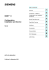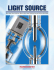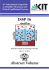# High-pressure Naphthalene-Xenon Phase Behavior

## Transcription

High-pressure Naphthalene-Xenon Phase Behavior
```Ind. Eng. Chem. Res. 1988,27, 1025-1033
1025
High-pressure Naphthalene-Xenon Phase Behavior
Mark A. McHugh,* James J. Watkins, and Brian T. Doyle
Department of Chemical Engineering, The Johns Hopkins University, Baltimore, Maryland 21218
Val J. Krukonis
Phasex Corporation, 360 Merrimack Street, Lawrence, Massachusetts 01843
The high-pressure phase behavior of the naphthalene-xenon system is experimentally determined.
Naphthalene solubility isotherms are obtained a t 34.9,40.0, and 45.0 "C over a range of pressures
from 105 to 270 bar. The pressure-temperature projection of the solid-liquid-gas (SLG) line is
obtained, and the upper critical end point (UCEP) is found a t 46.4 OC, 136.8 bar, and a naphthalene
mole fraction of 0.135. The location of the UCEP differs from a previously determined value; this
discrepancy is attributed to differences in the experimental techniques used to obtained the UCEP.
The phase diagram for the naphthalene-xenon system can be generated by using the Peng-Robinson
and the Sanchez-Lacombe equations of state each with two mixture parameters fit to the SLG line.
Although both equations represent the data with about the same accuracy, the Sanchez-Lacombe
equation does a better job fitting the SLG line and the observed liquid-gas and solid-gas phase
inversions.
In a previous paper (Krukonis et al., 1984),we reported
on the solubilities of naphthalene in supercritical xenon
(Tc = 16.7 OC, Pc = 58.8 bar) at temperatures of 34.9, 39.8,
and 45.0 OC and for pressures to 225 bar. We also presented preliminary data on the pressuretemperature (P-T)
trace of the xenon-naphthalene, solid-liquid-gas (SLG)
line. The results from that earlier study show that supercritical xenon has a solvent power which is comparable
to that of supercritical carbon dioxide, ethylene, and ethane. Other investigators have also corroborated this
finding.
Smith et al. (1987), who characterize the solvent power
of a supercritical fluid based on the T* polarity/polarizability scale established by Kamlet, Taft, and co-workers,
find that xenon has a solvent power that is better than that
of supercritical sulfur hexafluoride, is comparable to that
of supercritical ethane, but is not as good as that of supercritical carbon dioxide or ammonia. French and Novotony (1986) used xenon as the mobile phase for supercritical fluid chromatography/FTIR spectrometry investigations. They were able to solubilize heavy phenols and
aldehydes in supercritical xenon. It should be noted that,
although liquid xenon is also a good solvent (Everett and
Stageman, 1978; Marshall et al., 1983; Rentzepis and
Douglas, 1981), supercritical xenon exhibits much greater
solvent capacity.
In the present work, we add to our previously reported
solubility isotherms (34.9,39.8, and 45.0 "C), we also extend the SLG line to the upper critical end point (UCEP),
and we measure the portion of the critical-mixture curve
which intersects the UCEP. Figure 1shows schematically
the type of P-T behavior expected for binary mixtures of
naphthalene, a heavy, nonvolatile solid, and supercritical
xenon (Krukonis et al., 1984). The characteristics of this
class of phase behavior are that the critical temperature
of the fluid ( Tcl) is much lower than the normal melting
temperature of the solid (TMJ, the critical conditions of
the two components differ substantially, and the molecular
size, shape, structure, and intermolecular potential of the
two components are also usually very different. As shown
in Figure 1, the critical-mixture (L = G) curve is intersected by the SLG line in two locations: the UCEP and
the LCEP (i.e., the lower critical end point). Supercritical
fluid extraction of a solid occurs in the P-T region between
the two branches of the SLG line, since only solid-fluid
* Author t o whom
0888-5885/88/2627-1025\$01.50/0
behavior is observed in this region regardless of the system
pressure.
One objective of this study was to obtain the entire SLG
line starting at the normal melting point of naphthalene
(TMZ = 80.2 "C) and ending at the UCEP. Care was taken
in determining the location of the UCEP since various
types of phase transitions can occur near the SLG line
depending on the overall composition of the mixture (van
Welie and Diepen, 1961a). These phase transitions are
described in detail in the following section of this paper.
A t the UCEP, a vapor-liquid critical point occurs in the
presence of excess solid, and critical opalescence can be
observed-naphthalene-xenon mixtures appear reddish
orange. To further verify the location of the UCEP, we
measured the portion of the critical-mixture curve which
intersects the SLG line. The critical-mixture curve represents liquid vapor critical points for mixtures with
varying concentrations of naphthalene and xenon. Once
the SLG line was determined, more solid-solubility data
were obtained at 34.9, 39.8, and 45.0 OC. The other objective of this study was to show how the resultant phase
behavior can be represented by the Peng-Robinson
equation of state and the Sanchez-Lacombe equation of
state. The merits of each of these approaches are discussed.
+
Experimental Section
The experimental apparatus used in this study is described in detail elsewhere (McHugh et al., 1984a). A
schematic diagram of the experimental apparatus is shown
in Figure 2. The main component of the system is a
high-pressure, variable-volume view cell. Xenon is quantitatively transferred into the high-pressureview cell which
has been previously charged with a measured amount of
naphthalene. The naphthalene-xenon mixture, viewed
through a boroscope (Olympus, Model D100-048-000-90)
placed against a Pyrex window secured at one end of the
cell, is mixed by a glass-encased stirring bar activated by
a magnet located below the cell. The contents of the cell
can also be projected onto a video monitor (Panasonic,
Model BT-S1900N) using a video camera (Panasonic,
of using the video equipment is that the image of the cell
contents is enlarged several times and, hence, is easier to
analyze and interpret.
In contrast to the first-melting technique that was used
previously to determine the P-T trace of the SLG line
0 1988 American Chemical Society
1026 Ind. Eng. Chem. Res., Vol. 27, No. 6, 1988
e!
I \
7-
n.
1
1
cl
y2
Temperature
Figure 1. Schematic pressure-temperature (P-T ) diagram for a
mixture consisting of a heavy, nonvolatile solid and a light gas.
1
! ""Fll
2
/
!
Temperature
Figure 2. Schematic diagram of the experimental apparatus used
in this study.
(Krukonis et al., 1984),we determine the P-T trace of the
SLG line and the location of the UCEP using a more exacting technique (van Welie and Diepen, 1961a). After a
known amount of naphthalene and xenon is loaded in the
view cell, the mixture is compressed to a high pressure by
a movable piston fitted within the cell and is heated until
either two phases, liquid + vapor, or a single phase, liquid,
vapor, or fluid, exists in the cell. The type of single phase
can only be determined by crossing a phase boundary. The
high-pressure view cell is isobarically cooled very slowly,
and the type of phase transition is noted. Four different
types of transitions can be observed depending on the
overall mixture composition.
If a liquid and a vapor are initially present, and if, upon
isobaric cooling, a solid falls out of solution, an SLG point
is obtained. However, if all of the liquid in the cell disappears before solid is formed, the transition is a dew point
as shown in Figure 3A. If all of the vapor disappears
before solid is formed, the transition is a bubble point as
shown in Figure 3B. Notice that a critical point does not
occur for the constant-composition, P-T loop shown in
Figure 3A, because the loop is intersected by a solid-phase
region. But, a critical point does occur on the P-T loop
for the mixture shown in Figure 3B.
Finally, if only a single vapor or liquid phase is initially
present in the cell, and if, upon isobaric cooling, solid
precipitates from the solution without the formation of a
third phase, the temperature of the solid-fluid transition
can be either less than (Figure 3A) or greater than (Figure
3B) the SLG temperature. By use of the technique described in the previous paragraphs, the solubility of
naphthalene in supercritical xenon at the UCEP is obtained by repeating the SLG determination with differing
overall mixture compositions.
The critical-mixture curve is measured in the following
manner (Occhiogrosso et al., 1986). At a temperature
slightly higher than the UCEP temperature, a vapor-liquid
mixture at a fixed overall concentration is compressed to
Figure 3. Schematic P-T diagram showing the effect of composition
on the types of phase transitions which can occur near the upper
critical end point (UCEP). Part A shows the solid-liquid-gas (SLG)
line with a section of a constant-composition loop at a concentration
of heavy component which is less than that at the UCEP. Part B
shows the SLG line with a section of a constant-composition loop at
a concentration of heavy component which is greater than that at the
UCEP. The dotted portion of the SLG line leading to the UCEP is
not observable since the concentration is too high.
Table I. Pressure-Temperature Trace of the
Solid-Liquid-Gas Line for the Naphthalene-Xenon System
Obtainedin This Study
P, bar
T,"C
P, bar
T. "C
136.8
136.5
136.1
135.4
131.2
129.8
126.4
124.1
113.4
46.43"
46.59
46.43
46.72
46.35
46.39
46.13
46.69
47.10
108.2
108.2
102.8
102.8
100.1
96.5
94.1
88.3
79.8
48.3
47.44
47.81
47.34
47.96
48.00
48.10
48.10
49.00
51.50
63.20
UCEP, 13.5 mol 70 naphthalene.
a single phase. The pressure is then isothermally decreased
very slowly until the system becomes turbid and a second
phase just begins to precipitate. The transition is a critical-mixture point if critical opalescence is observed during
the transition process and if two phases of equal volume
are present when the mixture phase separates.
The reported pressures, measured with a bourdon-tube
Heise gauge, are accurate to within f0.34 bar, and the
reported temperatures, measured with a platinum RTD
(Degussa Company, calibrated on the 1968 IPTS scale),
are accurate to within hO.1 "C. The reported solid-solubility and critical-mixture data have an estimated, accumulated error which is less than 2.0% of the absolute value.
The naphthalene, obtained from Fischer Scientific (spectroscopic grade), was used without further purification, and
the xenon, donated by BOAC group, was also used without
further purification.
Results and Discussion
The P-T behavior of the naphthalene-xenon system is
shown in Figure 4 and is listed in Tables I and 11. The
Ind. Eng. Chem. Res., Vol. 27, No. 6, 1988 1027
0 A
along this portion of the line. The higher the solubility,
the larger the melting point depression of naphthalene.
Assuming that naphthalene and xenon form an ideal liquid
solution, the solubility of xenon in liquid naphthalene can
be estimated by using the following expression (De Swam
Arons and Diepen, 1963)
- Data obmned tn lhis study
i-DataafSeckner.etal.(19&1)
m. Data of Vargafuk (1975)
2
'
'
/
t
*'
20
60
40
TEMPERATURE
\"
80
(OC)
Figure 4. Experimentally determined pressure-temperature diagram for the naphthalene-xenon system obtained in this study.
Table 11. Pressure-Temperature-ConcentrationData for
the Section of the Critical-Mixture Curve Which Intersects
the UCEP
P, bar
T, O C
mol % naphthalene
139.2
49.6
13.48
144.1
53.6
13.48
148.8
57.1
13.48
152.9
60.2
13.48
P-T trace of the SLG line and the critical-mixture curve
is determined within f O . l "C and 0.5 bar. As shown in
Table 11, the concentration of naphthalene in supercritical
xenon remains essentially constant along the section of the
critical-mixture curve near the UCEP. This behavior has
been observed for other naphthalene-supercritical fluid
systems (van Hest and Diepen, 1960; van Welie and
Diepen, 1961b, 1963).
The UCEP for the naphthalene-xenon system is 46.4
f 0.1 "C, 136.8 f 0.5 bar, and 13.5 f 0.1 mol % naphthalene. The location of the UCEP is determined as the
intersection of the SLG line with the critical-mixture curve.
The low-pressure SLG data obtained by Krukonis et al.
(1984) are in good agreement with the data obtained in this
study. However, a t high pressures near the UCEP, the
first-melting technique used by Krukonis et al. leads to
spurious results as shown in Figure 4.
Also shown in Figure 4 is the vapor-pressure curve for
pure xenon (Vargaftik, 1975), which should be very close
to the branch of the SLG line which ends at the LCEP
(McHugh et al., 1984a,b; van Gunst et al., 1953). The
location of the LCEP, which is dependent on the solubility
level of naphthalene in xenon, is expected to be very close
to the critical point of pure xenon since the solubility of
a heavy solid in a supercritical fluid near its critical point
is usually quite low (McHugh and Krukonis, 1986).
The shape of the SLG line results from a compromise
between the effect of hydrostatic pressure on the melting
point of pure naphthalene, the solubility of xenon in the
naphthalene-rich liquid phase, and the solubility of
naphthalene in the xenon-rich gas phase (Paulaitis et al.,
1983). As seen in Figure 4, the initial slope of the SLG
line, starting at the melting point of naphthalene, is reasonably linear between 80 "C and l bar and 50 "C and 87
bar. For this section of the SLG line, the solubility of
naphthalene in the xenon-rich gas phase is expected to be
extremely low. Since the initial slope of the SLG line is
negative, the hydrostatic-pressure effect is dominated by
the solubility of xenon in the naphthalene-rich liquid phase
where TM2represents the normal melting temperature of
naphthalene (80.2 "C), Av represents the difference between the volume of pure, solid naphthalene and pure
subcooled liquid naphthalene (-18.7 cm3/mol (De Swaan
Arons and Diepen, 1963)), A H F is the heat of fusion of
naphthalene (4540 cal/mol (De Swaan Arons and Diepen,
1963)), and R is the gas constant. Using eq 1 with P-T
data from the initial slopes of their respective SLG lines,
it can easily be shown that xenon is more soluble in liquid
naphthalene than ethane (van Welie and Diepen, 1963),
ethylene (van Gunst et al., 19531, carbon dioxide (McHugh
and Yogan, 1984), and methane (van Hest and Diepen,
1960). It also follows that the melting point depression
of naphthalene caused by xenon, 34 "C, is greater than that
caused by ethane, 25 "C, ethylene, 29 "C, carbon dioxide,
20 "C, and methane, 5 OC.
At pressures greater than 87 bar, the solubility of xenon
in the naphthalene-rich liquid phase does not increase very
rapidly, and hence, the shape of the SLG line is a result
of a compromise between the effect of hydrostatic pressure,
which imparts a positive slope to the line, and the solubility
of naphthalene in supercritical xenon, which determines
the location of the UCEP. If the solubility of naphthalene
in supercritical xenon is moderately high, the UCEP will
occur at modest pressures very soon after the linear region
of the SLG line similar to what occurs with the naphthaleneethylene and naphthalene-ethane systems. However,
if the solubility of naphthalene is low, the UCEP does not
occur until very high pressures as with the naphthalenemethane system, or it does not occur at all as with the
helium-hydrogen system (Streett, 1973).
A number of interesting phase inversions occur with the
naphthalene-xenon system. At pressures above about 80
bar, the density of the naphthalene-rich liquid phase and
the xenon-rich gas phase along the SLG line becomes
greater than that of the pure, solid naphthalene phase.
Hence, solid naphthalene floats to the top of the view cell.
This behavior is quite interesting since the xenon-rich gas
phase is virutally pure xenon at these conditions and it is
somewhat unexpected that the gas phase would become
more dense than the solid phase. The solid-gas inversion
probably occurs because xenon is one of the more dense
supercritical fluids and it is very compressible. It is perhaps more unexpected that the addition of supercritical
xenon to liquid naphthalene would raise the density of the
resultant liquid mixture above that of the solid since
usually the addition of a gas to a liquid decreases the
density. However, as stated before, xenon is a very dense
gas which also has a molecular weight that is close to that
of naphthalene. It is conjectured that the solid-liquid
inversion occurs because the partial molar volumes of
xenon and naphthalene in the liquid phase are probably
less than their respective molar volumes at the same temperature and pressure.
If the pressure is increased to about 112 bar, the xenon-rich gas phase becomes more dense than the liquid
phase, and therefore, the gas phase settles to the bottom
of the cell with the liquid phase in the middle and the solid
phase at the top. The liquid-gas density inversion is also
1028 Ind. Eng. Chem. Res., Vol. 27, No. 6, 1988
Table 111. Solubility of Naphthalene in Supercritical
Xenon Obtained in This Study
naphthalene,
naphthalene,
P, bar
mol %
P. bar
mol %
T = 34.9 "C
189.1
4.53
T = 40.0
237.4
139.7
194.8
"C
7.52
4.53
7.24
T = 45.0 "C
105.5
2.36
159.6
129.4
4.53
166.1
133.0
7.24
171.6
135.9
5.76
175.2
141.2
7.69
177.7
1
o or
0 05
0 '0
" '5
143.2
10.33
181.1
152.3
8.30
260.3
\IO1 E FRACTIOY \IAPWITlALFhZ
153.6
11.32
268.0
Figure 5. Comparison of the solid-solubility data obtained in this
158.7
10.07
study (open symbols) with the data of Krukonis et al. (1984) (closed
symbols).
observed in the L + G region of the phase diagram at
pressures greater than 112 bar. At 80 bar, the gas phase
is probably greater than 99 mol % xenon, while at 112 bar
it has probably only been reduced to 98 mol % Since the
solubility of naphthalene in the gas phase increases by only
a small amount and since the operating condition is far
from the critical point of pure xenon, it seems unlikely that
the inversion is driven by a partial molar volume phenomenon. The liquid-gas inversion is probably a densityor pressure-driven phenomenon-that is, the gas phase is
still highly compressible, and thus, the density increases
by a significant amount as the pressure is increased. At
the same time, the concentration of the liquid phase has
not changed considerably and the liquid is not expected
to be very compressible. Therefore, the density of the
liquid should remain virtually unchanged as the pressure
is increased. Phase inversions have been observed in other
solute-supercritical fluid systems (McHugh and Yogan,
1984), and thus, it is very important from a processing
standpoint to be cognizant that these inversions can occur.
Listed in Table I11 and shown in Figure 5 are the
naphthalene-xenon solubility isotherms at 34.9,40.0, and
45.0 "C. A t 34.9 "C the solubility of naphthalene in supercritical xenon quickly reaches a limiting value of about
4.5 mol % at 200 bar. If the temperature is increased to
40.0 "C, the limiting solubility reaches about 7.5 mol 70
at 245 bar. However, if the temperature is raised 5 more
deg to 45.0 "C, the solubility isotherm shows an inflection
at about 135 bar where the solubility increases quite significantly for a small change in pressure. The inflection
in the solubility curve at 45.0 "C and 135 bar is caused by
the vapor-liquid critical point which occurs at the UCEP.
The scatter in the data for this isotherm is expected since
the solvent power of a supercritical fluid is very sensitive
to small changes in pressure and temeprature near a
critical-mixture point (McHugh and Krukonis, 1986).
Notice, also, that at 45.0 "C the solubility of naphthalene
in supercritical xenon continues to increase to values as
high as 13 mol % as the pressure is increased above 135
bar. Eventually a limiting solubility is reached at sufficiently high-enough pressures.
.
Data Reduction
The phase-equilibrium data obtained in this study are
modeled with the Peng-Robinson equation of state (Peng
and Robinson, 1976) and the Sanchez-Lacombe equation
of state (Sanchez and Lacombe, 1978). The Peng-Rob-
9.64
10.22
9.84
13.48
12.34
12.09
12.91
12.60
inson equation is a cubic equation which uses a van der
Waals type repulsive term with a modification of the van
der Waals attractive term, while the Sanchez-Lacombe
equation is a lattice-gas equation which uses a van der
Waals attractive term with a lattice-gas repulsive term.
Our objectives in this section are to show the merits of each
of these equations for calculating high-pressure phase
behavior and to describe how the phase diagram for solid-gas systems can be readily calculated by fitting the
parameters of the equation of state to the P-T trace of the
SLG line (McHugh et al., 1984a,b). A detailed development of the necessary equations for modeling the SLG line
is found elsewhere (McHugh and Krukonis, 1986) and is,
therefore, only briefly described here.
At equilibrium, the fugacities of each of the components
present in each of the phases must be equal. Although
there are four fugacity equalities along the SLG line, one
of the equations is redundant. We chose to solve the
following three equations:
FZG= F2
F2G = FzL
FIG = F 1
where F represents the fugacity, subscripts 2 and 1 represent naphthalene and xenon, and the superscripts S, L,
and G represent the solid, liquid, and gas phases, respectively. Solid naphthalene, with a density of 1.145 g/cm3,
will be considered as a pure solid which is incompressible
over the range of pressures of interest in this study (Vaidya
and Kennedy, 1971). Therefore, eq 2 becomes
where y 2 is the mole fraction of naphthalene,
is the
fugacity coefficient of naphthalene, P is the system presis the sublimation pressure of naphthalene, and
sure, PZsub
uZsis the molar volume of solid naphthalene.
Equations 3 and 4 can be written as
Y24ZG = 3C24zL
Y1hG
=
where x represents a liquid-phase mole fraction.
When the Peng-Robinson equation is used to determine
Ind. Eng. Chem. Res., Vol. 27, No. 6, 1988 1029
the fugacity coefficients, the following mixing rules are
used:
amix
= CCxixjaij
i
aij =
(8)
l
(a..a..)0.5(1
11 11
- k..)
11
bmix
(9)
= XZxixjbij
(10)
i l
bij = 0.5[(bii
+ bjj)(l - vij)]
(11)
where k..and qij are mixture parameters which are determinel by fitting the Peng-Robinson equation to the
SLG line and aii and bii are pure component parameters
as defined by Peng and Robinson (1976). With these
mixing rules, the expression for the fugacity coefficient
becomes
In
(bmkN)'
#i
=(2 - 1 ) - In (2 - B ) -
€mix
bmix
= (l/u*mi,)CC~i~j€iju*ij
i
"(
2.828B ( 2 C x j a i j ) , a - (bm"'bmix
)
In
(Zz +-
2.414B
0.414B)
(12)
where 2 is the mixture compressibility, N is the total
number of moles of the mixture, and A and B are pure
component parameters as defined by Peng and Robinson
(1976). (bmixN)'
is given as
N
(bmixN)'
equation to liquid molar volume data at 80-90 OC and over
a pressure range of 1 W 2 0 0 bar (Russell and Hottel, 1938)
and to VLE data (API Tables, 1980) in a temperature
range of 180-218 "C.The Sanchez-Lacombe equation was
also simultaneously fit to the normal melting point for
naphthalene when determining the characteristic parameters. If the melting point of naphthalene is neglected
when fitting pure component parameters, the calculated
melting point for naphthalene could be off by several
degrees. The characteristic parameters for xenon and
naphthalene are given in Table IV.
For naphthalene-xenon mixtures, the characteristic
temperature is defined as
T*mix = tmix/R
(16)
where tmk, the interactive energy for the mixture, is expressed as (Sanchez and Lacombe, 1978)
= i 3 ( b m i x N ) / d N ; = 2 C ~ k b i k- bmi,
k
Eij
(17)
J
= (tiitjj)o.5(1- kij)
(18)
The close-packed volume for the mixture, u * ~ is~given
~ ,
as
u* mix
. =
[email protected]@U*..
I
11
i j
1
~ * i=
j 0 . 5 [ ( ~ * i+i ~ * j j ) (-l vij)]
(19)
(20)
and \$i, the close-packed volume fraction of component i,
is defined as
(13)
Equation 13 is the corrected version of eq 5.17d given by
McHugh and Krukonis (1986). If qij is set equal to zero,
the mixing rule for bmk and the equation for the fugacity
coefficient reduces to the form given by Peng and Robinson (1976). The pure component characteristic properties
needed with the Peng-Robinson equation include the
critical temperature, the critical pressure, and the accentric
factor for each of the components.
The Sanchez-Lacombe equation of state, which is based
on lattice-fluid theory, is also used in this study. This
equation of state is
where m, is the weight fraction of component i. The
mixture parameter, kij, accounts for deviations of the
mixture interaction energy from the geometric mean of the
pure component energies, and qlj, a second mixture parameter, accounts for the deviation of the arithmetic average in the close-packed volume of the mixture.
The characteristic pressure of the mixture is given as
P*mix
RT*mix
=U*mix
And the number of lattice sites occupied by an rmer in the
mixture is given by
r = Zxir?
i
where P, p, and p are the reduced pressure, temperature,
and density, respectively. The parameter r represents the
number of lattice sites occupied by a molecule and is given
by
where M is the molecular weight and R is the gas constant.
For xenon, T*,Pr,and p*, the characteristic temperature,
pressure, and close-packed mass density, are obtained by
fitting the Sanchez-Lacombe equation to liquid molar
volume data at temperatures between 45 and 55 "C and
pressures between 80 and 150 bar (Michels et al., 1954) and
to the vapor-liquid equilibrium (VLE) line (Vargaftik,
1975) to within 11 "C of the critical temperature. The
pressure-temperature conditions chosen for fitting the
liquid molar volume data are in the vicinity of the UCEP
which is the most important P-T region for this study.
The fit to VLE data gives reliable values for T * and P,
while the fit to liquid molar volume data provides a reliable
value for p * . The pure component parameters for naphthalene are obtained by fitting the Sanchez-Lacombe
(23)
where xi represents the mole fraction of component i and
r? represents the number of sites occupied by a molecule
in the pure state.
Fugacity coefficients are obtained with the SanchezLacombe equation of state by using the following equation:
The expressions for the fugacity coefficient of the mixture
and for the derivative of the fugacity coefficient with respect to composition are given in the Appendix,
The fit of the SLG line using either the Peng-Robinson
or the Sanchez-Lacombe equation with a given estimate
of k and qlj was obtained in the following manner. A t a
fixe3 pressure and temperature, eq 5 was solved to determine the composition of naphthalene in the gas phase.
Next, eq 6 and 7 were solved by guessing a liquid-phase
composition and iterating until the equations were satisfied. The sum of the calculated liquid-phase mole fractions
was then checked. If this sum was greater than 1.0, the
temperature was adjusted downward slightly and the
1030 Ind. Eng. Chem. Res., Vol. 27, No. 6, 1988
Table IV. Characteristic Parameters for Xenon and
Naphthalene Used with the Sanchez-Lacombe Equation of
State
comDonent
D * . a/cm3
T*, K
PL,bar
xenon
3.040
354
2483
naphthalene
1.109
668
4543
3
PRESSURE (BAR)
40
45
5C
55
60
65
70
75
83
9
TEMPERATLRE ( C)
Figure 6. Comparison of calculated and experimental phase behavior. The solid lines are calculations with the Peng-Robinson
equation with k,, equal to 0.020 and ql, equal to -0.025. The dashed
lines are calculations with the Sanchez-Lacombe equation with k,,
equal to 0.030 44 and q,, equal to -0.0354.
calculational procedure was restarted at eq 5 using the
same pressure as before. If the sum of the liquid-phase
mole fractions is less than 1.0, the temperature is raised
slightly and again the calculational procedure is restarted
at eq 5 using the same pressure as before. If the sum is
equal to 1.0, the result is recorded as a point on the calculated SLG line, the pressure is increased, and the calculations are restarted at eq 5 . A vapor-liquid critical
point is predicted at the UCEP. Once the SLG curve is
fit and the values for k,, and qll are determined, the critical-mixture curve and the solid-solubility isotherms are
calculated. For both the Peng-Robinson and the Sanchez-Lacombe equations, we found that changing k , had
a large effect on the UCEP temperature and a smaller
effect on the UCEP pressure, while changing qcl had a large
effect on the UCEP pressure and a smaller effect on the
UCEP temperature.
Using the Peng-Robinson equation as described in the
previous paragraphs, it was not possible to fit the normal
melting point of naphthalene to within 2 "C. However,
if the value for bzz is multiplied by 0.9944, the melting
point could be determined within 0.1 "C. This correction
factor was used throughout the remainder of the calculations.
The fit of the SLG line and the calculated criticalmixture curve using the Peng-Robinson equation is shown
in Figure 6. With a value of 0.020 for k , and -0.025 for
qll, the calculated UCEP is 46.5 "C and 136.8 bar. As
shown in Figure 6, the calculated and experimental UCEP
are in good agreement, while the calculated SLG line is
in poor agreement with experimental data. A better fit
of the low-pressure range of the SLG line can be obtained
with different values of k,, and qkl;however, it is not possible to simultaneously fit the entire curve and the UCEP.
It was also not possible to obtain as good a fit of the UCEP
with q, set equal to zero. Dieters and Schneider (1976)
also found that two mixture parameters are needed with
the Redlich-Kwong equation of state to fit high-pressure
Figure 7. Comparison of calculated and experimental densities
along the solid-liquid-vapor line. The solid lines are calculations
with the Peng-Robinson equation with k,, equal to 0.020 and q,,
equal to -0.025. The dashed lines are calculations with the Sanchez-Lacombe equation with k, equal to 0.030 44 and qz, equal to
-0.0354.
phase behavior data. The calculated pressure projection
of the critical-mixture curve, which was determined using
the values of k,, and ql found from the fit of the SLG, is
in fair agreement with experimental data as shown in
Figure 6. The pressure along the calculated critical-mixture curve is consistently higher than the experimentally
observed data.
Figure 7 shows the calculated phase inversions which
occur along the SLG line. At pressures greater than 130
bar, the Peng-Robinson equation predicts that the gas
phase becomes more dense than the liquid phase. Experimentally the inversion of these phases occurs at about
112 bar. The calculated solid-gas inversion, 100 bar, is also
greater than the experimentally observed value of about
80 bar.
Calculated solid-solubility isotherms are shown in Figure
8. In general, the Peng-Robinson equation of state overpredicts the solubility of naphthalene in supercritical
xenon by about 10% at 35 "C and by 14-16% at 45 "C and
pressures near the UCEP. It is not surprising that the
predictions are off by such a large amount near the UCEP
since these operating conditions are very close to the vapor-liquid critical point which occurs at the UCEP. If k ,
and ql, are allowed to vary slightly, a much better fit of the
solubility isotherms could be obtained at the cost of a
poorer fit of the SLG line and the UCEP. Nevertheless,
by use of mixture parameters fit to the P-T of the SLG
line, a reasonable estimate can be made of the solubility
of the solid in the supercritical fluid.
The analogous phase equilibrium calculations made with
the Sanchez-Lacombe equation of state are also shown in
Figures 6-8. With a value of 0.030 44 for k , and -0.0354
for q,], the UCEP is calculated as 46.5 "C and 136.9 bar.
These values for k,] and q, are similar to those used with
the Peng-Robinson equation. As shown in Figure 6, the
fit of the SLG line is much better with the Sanchez-Lacombe equation than with the Peng-Robinson equation.
However, the pressure along the critical-mixture curve
calculated with the Sanchez-Lacombe equation was greater
than that of the data or the values calculated with the
Peng-Robinson equation.
Figure 7 shows that the phase inversions along the SLG
line calculated with the Sanchez-Lacombe equation were
in much better agreement with the experimental data than
Ind. Eng. Chem. Res., Vol. 27, No. 6, 1988 1031
I
found with the Peng-Robinson equation.
I
Conclusions
In this paper we have shown the utility of using a more
exacting technique for obtaining the P-T trace of the SLG
line ending at the UCEP. We have added experimental
information about the critical-mixture curve and solidsolubility isotherms for the xenon-naphthalene system.
And we have shown how a simple equation of state, either
the Peng-Robinson or the Sanchez-Lacombe, can be used
with a small amount of experimental data to generate
major portions of the P-I diagram, thus reducing the
amount of experimental effort needed to understand the
phase behavior for a particular solid-supercritical fluid
system.
Although both the Peng-Robinson and the SanchezLacombe equations represent the experimental data with
about the same accuracy, each of these equations has
different strengths. The Peng-Robinson equation uses the
critical properties of the pure components which, for very
large species, may not be available. The Sanchez-Lacombe
equation does not need critical properties to determine
pure component parameters but does need some VLE data
and liquid molar volume data. The main advantage with
the Sanchez-Lacombe equation is that is can be used for
large molecule systems, including polymer-solvent mixtures, whereas the Peng-Robinson equation is limited to
low-to-moderate molecular weight systems.
I
/
I
A -35'C
1
0 -45%
002
004
006
008
010
012
014
016
018
MOLE FRACTION NAPHTWALEW
Figure 8. Comparison of calculated and experimental solid-solubility isotherms. The data of Krukonis et al. (1984) are included on
this figure. The solid lines are calculations with the Peng-Robinson
equation with k,J equal to 0.020 and qlJequal to -0.025. The dashed
lines are calculations with the Sanchez-Lacombe equation with k,,
equal to 0.030 44 and qv equal to -0.0354.
were the results obtained with the Peng-Robinson equations. Figure 8 shows calculated solid-solubilityisotherms
using the mixture parameters found from the fit of the
SLG line. At 35 and 40 "C, the fit with the Sanchez-Lacombe equation is slightly better than that obtained with
the Peng-Robinson equation. At 45 "C, the fit of the data
is very similar for both equations up to about 175 bar. At
pressures above 175 bar, the solubilites calculated with the
Sanchez-Lacombe equation are about 25% too high.
The calculated phase behavior in Figures 6-8 shows that
a reasonable estimate for the phase behavior of a solid-gas
system can be obtained with either the Peng-Robinson or
the Sanchez-Lacombe equations of state, each with two
mixture parameters fitted to the SLG line. The P-T trace
of the SLG line is experimentally easier to obtain than
solid-solubility and critical-mixturedata, and it also defines
the P-T boundaries where supercritical fluid extraction
of a solid is possible. Hence, by fitting the mixture parameters in these equations to the "easier-to-obtain" data,
it is possible to generate reasonable estimates for the
solubility of a nonvolatile solid in a supercritical fluid as
well as other features of the phase diagram such as the
critical-mixture curve and the P-T conditions for phase
inversions.
Both equations of state are easy to implement; however,
they do differ in the amount of pure component information that is needed to determine the pure component
parameters used in the equations. The Peng-Robinson
equation uses critical property information, while the
Sanchez-Lacombe equation uses vapor-liquid equilibrium
data along with liquid molar-volume data. The PengRobinson equation is limited in use to low-to-moderate
molecular weight species for which critical-property information is available. The Sanchez-Lacombe equation
can be used to model the phase behavior of large molecular
weight species, including polymers and, hence, has a
greater utility for modeling the behavior of mixtures consisting of a low molecular weight gas and a large molecular
weight nonvolatile substance (Kiszka et al., 1988). Although more work needs to be done with the SanchezLacombe equation of state to correlate the mixture parameters, k, and vl,, to the pure component properties of
the mixture components, the results of this short study
suggest that k, and qL,should have values similar to those
Acknowledgment
We thank the Exxon Education Foundation for partial
support of this project and the BOAC Group who donated
the xenon used in this study. We also thank Melchior
Meilchen for helping with the calculations presented in
this paper. Mark McHugh especially thanks Rahoma
Mohamed, who pointed out the error in eq 5.17d in
McHugh and Krukonis (1986).
Nomenclature
F = fugacity
kI2 = mixture parameter
mi = weight fraction of component i
= pressure
P = reduced pressure
r = number of lattice sites occupied by a molecule
R = gas constant
T = temperature
T = reduced temperature
u = molar volume
ii = reduced volume
x = mole fraction
z = compressibility
e
Greek Symbols
e = interaction energy
vi,
p
= mixture parameter
= density
/? = reduced density
di = close-packed volume fraction of component i; fugacity
coefficient
Subscripts
C = critical property
i = component i
M2 = melting temperature of component 2
m,mix = mixture
Xe = xenon
1 = xenon
2 = naphthalene
1032 Ind. Eng. Chem. Res., Vol. 27, No. 6, 1988
Equations A3-A13 are substituted into eq 24 along with
values for the pure component parameters for each component.
Superscripts
F = fusion
G = gas
L = liquid
o = pure component
s = solid
sub = sublimation
* = characteristic variable
Registry No. Xe, 7440-63-3;
naphthalene, 91-20-3.
Literature Cited
Appendix. Determination of Fugacity
Coefficients Using the Sanchez-Lacombe
Equation of State
By definition, the fugacity coefficient of a mixture is
given as
In 4m =
Lm(A
-
d V + 2 - 1 - I n Z (Al)
where V is the total volume of the mixture and Z is the
compressibility factor which, in terms of the SanchezLacombe equation, is defined as
z = -rPii
(-42)
T
The natural log of the fugacity coefficient of the mixture
is obtained by substituting the Sanchez-Lacombe equation
of state into eq Al:
ln\$,={B-1)rln
+r+Z-l-lnZ
(A3)
Equation A3 is then substituted into eq 24. The following
expression was derived for In 4, by using the chain rule
of differentiation,
In 4 m --a In 4, aa a In 4, ar
-ax1
ao ax,
ar ax,
a-ln 4, a In 4,
In 4, az
+
-- (A4)
aT
ax,
az ax,
where the derivatives in eq A4 are
+--
ar
+
API Tables, Research Project 44,loosefleaf supplements to 1980;
American Petroleum Institute and the Thermodynamics Research
Center, Texas A&M University, College Station, TX.
Dieters, U.; Schneider, G. M. “Fluid Mixtures at High pressures:
Computer Calculations of the Phase Equilibria and the Critical
Phenomena in Fluid Binary Mixtures from the Redlich-Kwong
Equation of State”. Ber. Bunsenges. Phys. Chem. 1976,80,1316.
De Swaan Arons, J.; Diepen, G. A. M. “Thermodynamic Study of
Melting Equilibria under Pressure of a Supercritical Gas”. Recl.
Trau. Chim. Pays-Bas 1963,82,249.
Everett, D. H.; Stageman, J. F. “Preparation and Stability of Novel
Polymer Colloids in a Range of Simple Liquids”. Faraday Discuss. Chem. SOC.1978,65,230.
French, S. B.; Novotony, M. “Xenon, a Unique Mobile Phase for
Supercritical Fluid Chromatography”. Anal. Chem. 1986,58,164.
Kiszka, M. B.; Meilchen, M.; McHugh, M. A. “Modeling HighPressure Gas-Polymer Mixtures Using the Sanchez-Lacombe
Equation of State“. J. Appl. Polym. Sci. 1988,in press.
Krukonis, V. J.; McHugh, M. A.; Seckner, A. J. “Xenon as a Supercritical Solvent”. J. Phys. Chem. 1984,88,2687.
Lacombe, R. H.; Sanchez, I. C. “Statistical Thermodynamics of Fluid
Mixtures”. J. Phys. Chem. 1976,80, 2568.
Marshall, D. B.; Strohbusch, F.; Eying, E. M. ‘Solubility of Organic
Substances in Liquid Xenon”. J. Chem. Eng. Data 1983,26,333.
McHugh, M. A,; Krukonis, V. J. Supercritical Fluid Extraction:
Principles and Practice; Butterworth Stoneham, MA, 1986;
Chapter 3.
McHugh, M. A.; Yogan, T. J. “A Study of Three Phase Solid-Liquid-Gas Equilibria for Three Carbon Dioxide-Solid Hydrocarbon
Systems, Two Ethane-Hydrocarbon Solid Systems and Two
Ethylene-Hydrocarbon Solid Systems”. J. Chem. Eng. Data
1984,29, 112.
McHugh, M. A,; Seckner, A. J.; Yogan, T. J. “High-pressure Phase
Behavior of Octacosane and Carbon Dioxide”. Ind. Eng. Chem.
Fundam. 1984a,23,493.
McHugh, M. A.; Seckner, A. J.; Krukonis, V. J. “Supercritical
Xenon”. Presented a t the AIChE Meeting, San Francisco, CA,
Nov 198413.
Michels, A.; Wassenaar, T.; Louwerse, P. “Isotherms of Xe”. Physicu
1954,20,99.
Occhiogrosso, R. N.;Igel, J. T.; McHugh, M. A. “The Phase Behavior
of Isopropylbenzene-Cop Mixtures”. Fluid Phase Equilib. 1986,
26,1088.
Paulaitis, M. E.; McHugh, M. A,; Chai, C. P. “Solid Solubilities in
Supercritical Fluids a t Elevated Pressures”. In Chemical Engineering at Supercritical Fluid Conditions; Paulaitis, M. E., Penninger, J. M. L., Gray, R. D., Davidson, P., Eds.; Ann Arbor Science: Ann Arbor, MI, 1983;p 139.
Peng, D.-Y.; Robinson, D. B. “A New Two Constant Equation of
State”. Ind. Eng. Chem. Fundam. 1976,15,59.
Rentzepis, P. M.; Douglas, D. C. “Xenon as a Solvent”. Nature
(London) 1981,293,165.
Russell, F. R.; Hottel, H. C. “Compressibility of Liquid
Naphthalene”. Znd. Eng. Chem. 1938,30,343.
Sanchez, I. C.; Lacombe, R. H. “Statistical Thermodynamics of
Polymer Solutions”. Macromolecules 1978,6,1145.
Smith, R. D.; Frye, S. L.; Yonker, C. R.; Gale, R. W. “Solvent
Properties of Supercritical Xe and SF,”. J.Phys. Chem. 1987,91,
3059.
Streett, W. E. “Phase Equilibria in Molecular Hydrogen-Helium
Mixtures at High Pressure”. Astrophys. J. 1973,186, 1107.
Vaidya, S. N.; Kennedy, G. C. “Compressibility of 18 Molecular
Organic Solids to 45 kbar”. J. Chem. Phys. 1971,55,987.
van Gunst, C. A,; Scheffer, F. E. C.; Diepen, G. A. M. “On Critical
Phenomena of Saturated Solutions in Binary Systems. 11”. J .
Phys. Chem. 1953,57,578.
van Hest, J. A. M.; Diepen, G. A. M. “Solubility of Naphthalene in
Supercritical Methane”. Symp. Soc. Chem. Ind. London 1960,lO.
Ind. Eng. Chem. Res. 1988,27, 1033-1041
van Welie, G. S. A.; Diepen, G. A. M. "The P-T-x Space Model of
the System Ethylene-Naphthalene (I)*. Red. Trau. Chim.
Pays-Bas 1961a,80,659.
van Welie, G. S. A.; Diepen, G. A. M. " P-T-x Space Model of the
System Ethylene-Naphthalene (111)". R e d . Trau. Chim. PuysBas 1961b,80,673.
van Welie, G. S. A.; Diepen, G. A. M. "The Solubility of Naphthalene
1033
in Supercritical Ethane". J. Phys. Chem. 1963,67, 755.
Vargaftik, N. B. Handbook of Physical Properties of Liquids and
Gases, Pure Substances and Mixtures, 2nd ed.; Hemisphere:
Washington, D.C., 1975; p 580.
Received for review September 17, 1987
Accepted January 27, 1988
Prediction of Solvent Activities in Polymer Solutions Using an
Empirical Free Volume Correction
Michael J. Misovich and Eric A. Grulke*
Department of Chemical Engineering, Michigan State University, 173 Engineering Building, East Lansing,
Michigan 48824 - 1226
A recent correlation for solvent activities in polymer solutions is extended in scope to provide a
methodology for modeling nonideal effects in polymer solutions. This new method allows the use
of any expression for the residual (enthalpic) interaction between polymer and solvent in conjunction
with a standard (Flory-Huggins) expression for the combinatorial entropy. An empirical free volume
correction uses the infinite dilution weight fraction activity coefficient of the solvent as an adjustable
parameter. T h e new method is applied using one residual term given by the Analytical Solution
of Groups (ASOG) technique, one similar to the Flory-Huggins interaction term, and one which
yields no residual interaction. The results of these three models are compared to one another and
to the Flory-Huggins model for 21 isothermal binary polymer-solvent systems. When adjustable
parameters are determined by best fit to the data, each of the models applying the new method results
in a standard error of less than 5 % for a t least 16 of the systems studied. This represented a better
performance than the Flory-Huggins model.
An understanding of the thermodynamics of polymer
solutions is important in practical applications such as
polymerization, devolatilization, and the incorporation of
plasticizers and other additives. Diffusion phenomena in
polymer melts and solutions are strongly affected by
nonideal solution behavior, since chemical potential rather
than concentration provides the driving force for diffusion.
Proper design and engineering of many polymer processes
depend greatly upon accurate modeling of thermodynamic
parameters such as solvent activities.
This work was an extension of previous work by the
authors for correlating solvent activities in polymer solutions (Misovich et al., 1985). In that paper, an empirical
free volume correction is derived from an athermal form
of the Flory-Huggins combinatorial entropy (Flory, 1953),
suggested by the Analytical Solution of Groups (ASOG)
group-contribution model for calculation of activity coefficients in solution (Derr and Deal, 1969). The technique
generally performs better than the classical Flory-Huggins
equation in extrapolating solvent activity data from low
solvent concentrations to higher concentrations. One deficiency of the approach is that phase separation cannot
be predicted; i.e., dal/dwl > 0 is always the case.
In this paper, the empirical free volume correction was
modified to allow the explicit inclusion of an expression
for residual (enthalpic) interaction between polymer and
solvent. A general scheme was given to accomplish this,
and three specific cases were analyzed and compared. One
case used the ASOG expression for residual interaction,
while a second used an interaction parameter approach
similar to the Flory-Huggins equation. The third case
assumed that there was no residual interaction term and
reduced to the generalized correlation previously cited
(Misovich et al., 1985).
The results in this paper were based upon a best fit of
the adjustable parameters in each model using a leastsquares evaluation of all the data, not by extrapolation
from a single data point. In each of the three cases, the
infinite dilution weight fraction solvent activity coefficient,
film,is an adjustable binary parameter. A residual interaction parameter is a second adjustable binary parameter
in the second case. The classical Flory-Huggins equation
was also fit to the data for comparison. In general, regardless of which residual interaction expression was used,
the new method fits the data with less error than the
Flory-Huggins equation.
Generalized Thermodynamic Modeling
Nonideal interactions between molecules in solution are
generally classified in one of two categories. Interactions
resulting from differences in the size or shape of molecules
are classified as entropic, while interactions resulting from
differences in energy are classified as enthalpic. The
complete expression for solvent activity, al, is typically
derived by multiplying concentration (mole fraction), xl,
a size or entropy activity coefficient, ylS, and a enthalpy
or group interaction activity coefficient, ylG,or by adding
their logarithms as shown in eq la. It is also common to
lump the concentration with one of the activity coefficients
(usually the entropic coefficient) to give eq lb.
In al = In x1 + In ylS + In ylG
(la)
In al = In alS + In ylG
(Ib)
A statistical approach allows entropic interactions to be
handled combinatorially, as is done by the athermal Flory-Huggins equation (Flory, 1953),giving for the entropic
contribution to activity, ulS
In alS = In (xlyls) = 1 - 41+ In 41
(2)
where x1 is the mole fraction, ylS is the entropic activity
coefficient, and c \$ ~ is the volume or segment fraction of
component 1 (solvent). Staverman (1950) has also given
an expression for combinatorial entropy which includes
0888-5885I88 12627-1033%01.50/0 0 1988 American Chemical Societv
```

### to the Xenon end-user brochure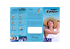### ri-scope® LED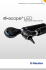### p - SAFIR2014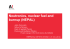### SUNTEST Family Brochure, English - In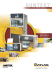### 1 SYLLABUS Course: General Chemistry II: CHEM-1100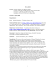### From the Principal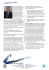### Numerical analysis of the influence of wall thermal inertia on the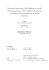### Development of a Pilot Scale Coal Powered Oxy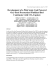### Siemens MOBY_D System Manual